# Mathematics

Advanced Calculator for school or study that allows you to calculate formulas, solve equations or plot functions.

FEATURES

Calculate any formula you want and show them in a 2d or 3d plot. The natural display shows fractions, roots and exponents as you would expect it from mathematics.

In a few seconds you derivate or integrate your desired function, calculate the zero points of your function und show them in the function plot. See all maxima, minima or inflection points in one view.

The easy way of use allows you to solve linear equations in just a moment. Or transform your mathematical, physical or chemical equation to any unknown variable.

You often needs to calculate with binary, octal or hexadecimal number systems? No problem! You can mix them together in one calculation even by using decimal digits. But that's not enough! You can also calculate with any other number system with base 2 to 18.

From time to time you may need to convert units to another one, like Celsius to Fahrenheit, miles to kilometre, inches to foot and so on.

You will also be able to calculate with vectors, matrices and determinants.

All this features are combined in this app and will make your mathematical life a lot easier.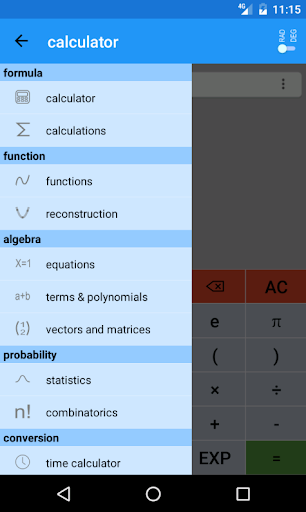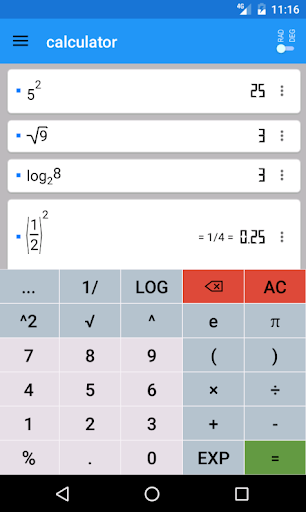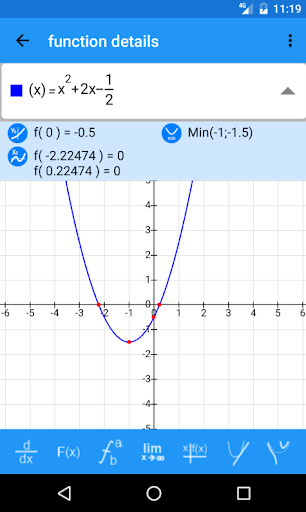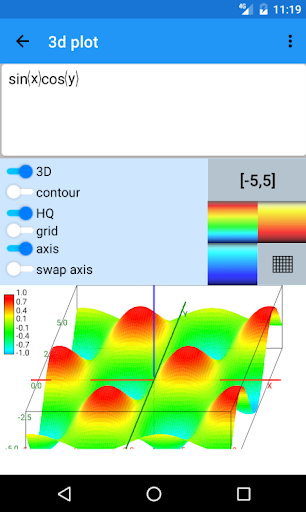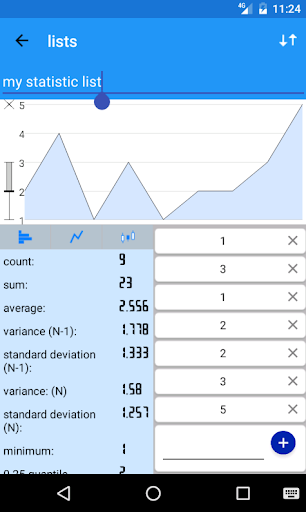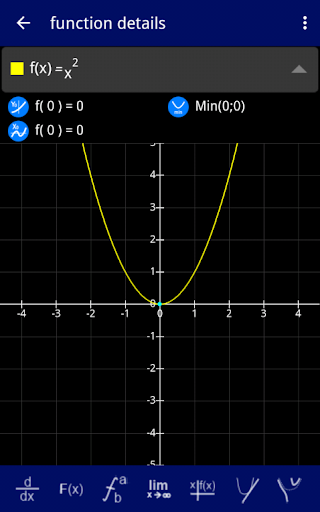##### Recent Updates - Version 3.4.3
• - average plot for statistic lists
- delete all values of statistic lists
- bugfixes